# Artificial Neuron Network Based Faults Detection and Localization in the High Voltage Transmission Lines with Mho Distance Relay

Artificial Neuron Network Based Faults Detection and Localization in the High Voltage Transmission Lines with Mho Distance Relay
Research Laboratory on the Electrification of Industrial Enterprises, University of Boumerdès, 35000, Algeria

Research Laboratory on the Electrical Engineering, Faculty of Technology, University of M’Sila, 28000, Algeria

Corresponding Author Email:
djalaleddine.khodja@univ-msila.dz
Page:
137-147
|
DOI:
https://doi.org/10.18280/jesa.530117
19 October 2019
|
Accepted:
13 December 2019
|
Published:
29 February 2020
| Citation

OPEN ACCESS

Abstract:

This study offers the opportunity to extend the functioning of the most advanced protection systems. The faults which can arise on the power transmission lines are numerous and varied: Short-circuit; Overvoltage; Overloads, etc. In the context of short circuits, the conventional sensor as the Mho distance relay also known as the admittance relay is generally used. This relay will be discussed later in this study. By taking into account the preventive risks of the Mho relay and discover the new techniques of artificial intelligence, namely the neural network which can contribute to the precise and rapid detection of all types of short-circuit faults. The results of the simulation tests demonstrate the effectiveness of the methods proposed for the automatic diagnosis of faults.

Keywords:

fault detection and localization, diagnosis, high voltage transmission, Mho distance relay, artificial neural network

1. Introduction

Electric transmission lines are subject to faults and malfunctions. The classification of these faults in electrical networks is an important problem. Many methods have been adopted to fulfill the task of diagnosing faults . Some methods used in this area include: Support Vector Machine (SVM) for fault diagnosis [1-3]. SVM is based on shape recognition algorithms. The goal is to classify the type of fault in the rows.

SVMs are used as an intelligence tool to identify the faulty line of the substation with the location of the distance . In addition, SVMs are compared to neural networks with basic radial functions in data sets corresponding to different defects. In the paper , the current and the voltage of each phase are sampled, calculated and then used as an optimal learning model. This method can identify each class with precision compared to certain previously used methods such as: expert system, Petri net and fuzzy theory .

Remote protection of flexible transmission lines, including thyristor controlled serial compensator (TCSC). This article  presents a new approach for the protection of the TCSC line using a support vector machine (SVM). The proposed method uses default current samples for half cycle (ten samples) from the creation of the fault angle and the trigger angle as inputs to the SVM.

The detection time and the location of the fault are determined on the basis of rules setting obtained from the current waveform analysis in the time and wavelet domains. The method is capable of distinguishing faults from other disturbances in the quality of energy, such as voltage dips and oscillatory transients, which are common in the operation of power systems [5, 6].

The wavelet packet transformation (WPT) is applied to the instantaneous voltage signals . The instantaneous active power components are obtained by multiplying the instantaneous currents obtained from a voltage source with these WPT based voltage signal components. A new feature vector extraction scheme is used in computing the energies of the instantaneous active power components.

These works propose a method for diagnosing faults in high-voltage lines based on neural modules. The latter take into account the fault voltage and current oscillograms as well as the frequency spectra of these oscillograms, in order to check whether the transmission line has been subjected to a fault [8-10].

Other works have applied technology that uses partial crown discharge detection to find faulty conditions while simultaneously performing routine visual inspections by a helicopter .

Furthermore, the diagnosis of faults in the high voltage transmission line (HTL) is a key research subject in the field of fault diagnosis of the electrical system. HTL fault diagnosis systems mainly depend on real-time information on the circumstances in the event of an accident. After the fault of HTL has been recognized, a series of methods of rapid restoration of the power system can be taken [12, 13].

High voltage transmission plays an important role in the fast restoration of the system. The fault diagnosis of a high-voltage transmission line involves three main tasks, namely the identification of the type of fault, the location of the faults and the estimate of the failure time .

The location of faults and the detection of a high-voltage transmission line are of paramount importance from the point of view of energy management services [14-16]. The fault types of the power system, i.e. single-phase ground faults, phase-to-phase faults, phase-to-earth faults, and ground are responsible for the transients of current and voltage waveforms in power systems food. Waveform coupling deals with the approximate overlap of these discrete waveforms obtained from recording devices and software [17, 18].

Constructed feature vectors are processed with a classifier for short-circuit faults occurring in high-voltage power lines; this is called the common vector approach (CVA). This is the first implementation of CVA analysis in the classification of short-circuit faults occurring in high-voltage power transmission lines. It is concluded that a combination of the proposed characteristic extraction scheme with CVA classifier gives significantly higher performance for short circuit classification defects in the transmission line .

This paper uses the Electromagnetic Transient Program (EMTP) for modeling an actual transmission system and MATLAB for Neural Network. Different types of defects have been simulated at different locations along the transmission line and an attempt has been made to correctly identify and locate the defect .

In this context of short circuits, the conventional sensor known as Mho relays is usually used. In fact, a Mho relay is a high-speed relay and is also known as the admittance relay. In this relay, the operating point is obtained by the volt-ampere element and the monitoring element is developed due to the voltage element. This means that the Mho relay is a directional relay with voltage control. This relay will be discussed later in this study.

This relay is limited in its use in the system of protection and prevention against random and unexpected defects of distribution networks (short circuit). The Mho relay is used to locate the fault, i.e. the fault at the distance from the event location that contributed to the failure of the power transmission "it is defined as a fault remote relay".

It offers the advantage of defining the zonal protection location:

• Zone 1 protected 80% of the line.

• Zone 2 protected 120% of the line.

• Zone 3 protected 160% of the line.

View the preventive hazards by the relay Mho and seen the new techniques of artificial intelligence namely artificial neurons networks. These can contribute to the accurate and rapid detection of all types of known short-circuit faults . As part of the development of this study, this work is articulated on the following sections: Section 2: protection of the HV line by the conventional relay Mho. Section 3: defects protection by neuronal relay. Section 4, the results of the simulation tests demonstrates the effectiveness of the proposed method for automatic fault diagnosis.

2. Basic Concepts in Protection Systems

Electrical protection systems are complicated systems that cannot be designed to protect the entire transportation system or electrical energy. When building the protection systems, the electrical network is divided into demarcated areas covered by the circuit breakers (see Figure 1). Each zone must be properly protected. The zones overlap so as not to leave any point of the network without protection.

Figure 1 illustrates the different protection zones of power transmission lines . The recovery area is distributed as follows:

• Zones 1 and 7: Protection relays are available which are responsible for the fault that may occur inside the generator.

• Zones 2 and 6: Protection relays are available which are responsible for all faults in the transformer.

• Zone 3 and 5: Protective relays are available for the protection of all faults found in bus bars.

For zone 4 relays: Responsible for all faults on power transmission lines.

It should be noted that the protection in each zone is actually made up of several protective devices and not a single device to detect all types of faults. There is an overlap between the different protection zones to ensure the possible case of unprotected areas, in particular the bar zone.

## 1.png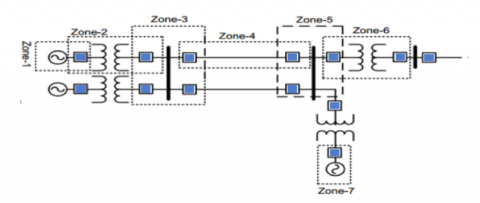Figure 1. Distribution of protection zones of an electricity network

2.1 Fault signal (Relaying signal)

When a malfunction is occurred, the voltage and current value change . The signal used in fault detection is called Relaying Signal. Among other things, the examples of faults encountered are:

• Frequency variation: (may increase or decrease).

• Direction of electric current can reverse the direction of the current, especially in the generators case.

• Impedance value Z (can change when the fault zones   change, this can help to detect the position of the latter).

• The difference between the input current value and the output current to an element (the large variation between them is the source of a fault).

A change in any of these signals (or in the voltage and current values) above allowable limits means that something abnormal has occurred in the network and must be discovered and studied, and subsequently isolate the network fault .

The main protection system of a zone can also become a back-up protection system in other zones, provided that the coordination rules between the different protection systems that make the emergency protection functional after some time (see Figure 2). This is allowed after confirmation of the failure of the main protection to detect faults .

## 2.pngFigure 2. Directional protection

Directional protection is a type of electrical protection that uses the direction of current or the flow of power, active or reactive, to determine if the protected area suffers a fault when one of these three values exceeds a threshold and that the direction is abnormal, the protection triggers. It is used to protect lines, alternators, transformers [6, 11]. Otherwise, the protection must be:

• Selective: To ensure discrimination of the faulty element.

• Safe: It must work in all the required cases.

• Autonomous: Safe power supply because it must operate in conditions of unfavorable power supply.

• Fast: It should work in as short a time as possible.

• Independent of the network configuration.

• Unaffected by variations in the network topology.

• Sensitive: It must work regardless of the intensity value, the nature and the location of the defect. Insensitive to allowable overloads and oscillations of U and I when walking out of sync to avoid a long and painful recovery of service .

2.2 Protection of the HV line by the conventional Mho relay

The distance protections are intended to protect the high-voltage lines against short circuits. The curve of variation of the short-circuit currents as a function of the distance of the fault perfectly shows the advantages offered by the time-distance characteristic of the protection such as:

- The speed of elimination of defects in the first zone which results in an appreciable reduction of investments.

- The backup protection function provided in the second and third zone for the bars and links of the opposite station.

In this section, we propose the simulation of the Mho relay for the protection of a HV transmission line against short-circuit currents [23, 24].

## 3.pngFigure 3. Installation diagrams of the equipment related to the remote protection

As for the relay of Mho, supplied locally by measurement reducers which provide the image of the network, in case of presence of electrical fault in its surveillance zone, the role of a protection is to detect it and to control the circuit breaker that will eliminate the fault.

The most sophisticated protections have a measurement by type of defect and use criteria allowing the validation of the measurement corresponding to the real defect. These are the multi-channel measurement protections, most often with six channels, used on 220 and 400 kV networks. Figure 3 illustrate the principle of installing different equipment related to remote protection [23, 24].

According to the measurement zones the remote protection is of four types namely: Mho, Circular, Polygonal and Elliptical. The curves below represent the respective characteristics at the four relays [24, 25].

It is worth mentioning the advantage offered by using the polygonal and elliptical characteristics especially when it comes to protecting the long or medium high-load electrical lines for which the service impedance can satisfy with the control impedance of the start of distance protection relays. Mho and circular characteristics are generally used to protect short HT lines that are not heavily charged [24, 25].

2.3 Principle of impedance measurement

Remote protection uses a distance measurement between the fault and the point where it is installed to locate a fault. It is determined by a measurement of the direct impedance, which varies from 0.33 Ω to 0.42 Ω per kilometer depending on the type of high-voltage line. This measure must have a directed character. Taking into account the reactive part of the direct impedance (Z_d) makes it possible to offset the distance measurement and the fault resistance . In the absence of a fault (see Figure 4), the distance protection sees only an apparent impedance of the network, dependent on the transit carried out on the line, but in no way comparable to the direct impedance of the line.

## 4.jpg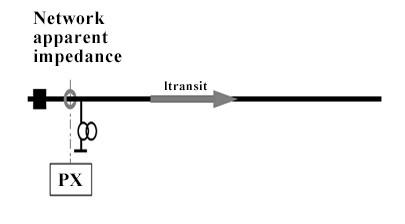Figure 4. Remote protection in the absence of a fault

The remote protection must therefore separate the situation with default from the normal situation without flaws: this is one of the roles of the start-up. In the presence of a fault (see Figure 5), the direct impedance between the fault point and the point where the protection is located can be determined from voltages and currents [23, 24].

## 5.png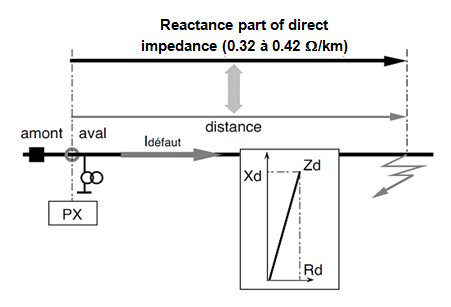Figure 5. Remote protection in the presence of a fault

2.4 Protection zone settings (Relationship between time and distance)

Most of the global distance protection relays are set for three downstream protection zones (Z1, Z2 and Z3) and one upstream protection zone (Z4) as shown in Figure 6 with selectivity well respected time clock for each zone [23, 24].

With regard to the adjustment of the downstream zones (see Figure 7), the zone Z_1 is set between 80 to 90% of the total impedance of the protected line AB (Z_AB) with an instantaneous time (T_1 = 0 sec) of tripping circuit breaker HT.

## 6.jpg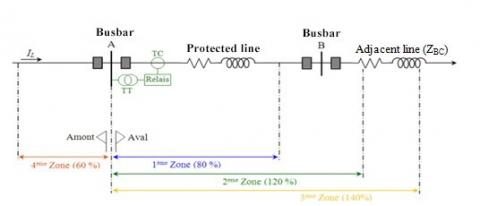Figure 6. Principle settings of the protection zones

## 7.jpgFigure 7. Chronometric selectivity

Zone Z2 must take into consideration the total impedance of the protected line (100% 〖Z〗_AB) plus part of the total impedance of the adjacent line (20% of Z_BC), with a delayed tripping time (T_2 = 0, 3 sec) .

This zone is an emergency zone for the remote relay installed on the adjacent line BC, it is characterized by:

- A desired minimum operating parameter which is the impedance at the remote bus bar multiplied by a safety factor of 1. 2.

- A minimum delay time associated with each distance relay installed which must coordinate with the T_2 zone of all its relays.

Zone Z3 must consider the total impedance of the protected line (100% of 〖Z〗_AB) plus part of the total impedance of the adjacent BC line (40% of 〖Z〗_BC), with a delayed tripping time (T_3 = 1.5 sec). At the same time, it is a backup zone for the distance relay installed on the adjacent line BC.

For upstream zones, the Z_4 zone must protect the AB line if the direction of transit power between the bus bars (from B to A) changes. It is set at 60% of the total impedance Z_AB, with a delayed tripping time (T_4 = 0.6 sec). The equations for calculating the zone settings are [23, 24]:

First Zone $\left(Z_{1}\right)$

$Z_{1}=80 \% Z_{A B}=0.8\left[\mathrm{R}_{\mathrm{AB}}+j . X_{A B}\right]$

Second Zone $\left(Z_{2}\right)$

$Z_{2}=Z_{A B}+20 \% Z_{B C}=\left[\mathrm{R}_{\mathrm{AB}}+\mathrm{j} \cdot X_{A B}\right]+0.2\left[\mathrm{R}_{\mathrm{BC}}+\mathrm{j} \cdot X_{B C}\right]$

Third Zone $\left(Z_{3}\right)$

$Z_{3}=Z_{A B}+40 \% Z_{B C}=\left[\mathrm{R}_{\mathrm{AB}}+j . X_{A B}\right]+0.4\left[\mathrm{R}_{\mathrm{BC}}+j . X_{B C}\right]$

Forth Zone $\left(Z_{4}\right)$

$Z_{1}=-60 \% Z_{A B}=-0.6\left[\mathrm{R}_{\mathrm{AB}}+\mathrm{j} . X_{A B}\right]$

In fact, for relay input signals, on a three-phase power system, there are ten distinct types of possible faults: a three-phase fault, three phase-phase faults, three-phase phase faults and three faults, double phase-ground. The equations governing the relationship between voltages and currents at the relay location are different for each of these defects. In the following Table 1, we will see the fault impedance algorithm for the different types of defects [26, 27]:

Table 1. The algorithm of faulty impedance

 Types of faults Algorithm AB or ABG $\left(\mathrm{V}_{\mathrm{A}}-\mathrm{V}_{\mathrm{B}}\right) /\left(\mathrm{I}_{\mathrm{A}}-\mathrm{I}_{\mathrm{B}}\right)$(1) AC or ACG $\left(V_{A}-V_{C}\right) /\left(I_{A}-I_{C}\right)$(2) BC or BCG $\left(V_{B}-V_{C}\right) /\left(I_{B}-I_{C}\right)$(3) AG $\mathrm{V}_{\mathrm{A}} /\left(\mathrm{I}_{\mathrm{A}}+3 \mathrm{K}_{0} \mathrm{I}_{0}\right)$(4) BG $\mathrm{V}_{\mathrm{B}} /\left(\mathrm{I}_{\mathrm{B}}+3 \mathrm{K}_{0} \mathrm{I}_{0}\right)$(5) CG $\mathrm{V}_{\mathrm{C}} /\left(\mathrm{I}_{\mathrm{C}}+3 \mathrm{K}_{0} \mathrm{I}_{0}\right)$(6) ABC or ABCG VA / IA= VB / IB = VC / IC

With:

A, B and C indicate defective phases, G indicates ground fault.

$V_{A}, V_{B} \cdot e t \cdot V_{C}$ indicate phase voltages. $\mathrm{I}_{\mathrm{A},} \mathrm{I}_{\mathrm{B}}$ and $\mathrm{I}_{\mathrm{C}}$ indicate the currents of the phases.

$Z_{0}$ = Homopolar impedance of the line.

$Z_{1}$  = Positive sequence impedance (direct) of the line.

K0 = Residual compensation factor where:

$\mathrm{K}_{0}=\left(\mathrm{Z}_{0}-\mathrm{Z}_{1}\right) / \mathrm{K} \cdot \mathrm{Z}_{1}$ can be 1 or 3 depend on the relay design.

In fact, relays are elements whose operation is based on the comparison of two independent quantities are essentially either amplitude or phase comparators [23, 28].

2.5 Protection features of distance Mho relay

The parameters of the composite signals in a comparator determine the shape, size and position of the operating characteristic in the impedance plane. The operating characteristics of the distance relays are generally geometric figures such as circles, straight lines or combinations thereof. However, in digital relays, it is possible to design operating characteristics of almost any shape. The most common operating characteristics used by distance relays are impedance, offset impedance, Mho, reactance, and quadrilateral characteristics. There are methods used to obtain different operating characteristics by the phase and amplitude comparators, special attention will be on Mho feature as it is widely used .

2.5.1 Characteristic of Mho phase comparator

A phase comparator checks the difference between the phase angles of the two composite signals and operates if the difference is within a specified range. The composite signals in a phase comparator are designated S1 and S2. An angular displacement is considered positive if S_1conduit S_2. A phase comparator works, if the following condition is satisfied:

$-90^{\circ} \leq \theta_{S 1}-\theta_{S 2} \leq \cdot 90^{\circ}$       (1)

The phase comparison signals S_1 and S_2 for producing the Mho characteristic are defined as follows [24, 25]:

$\mathrm{S}_{1}=-\mathrm{V}_{\mathrm{r}} \angle 0^{\circ}+\mathrm{Z}_{\mathrm{R}} \angle \theta^{\circ} * \mathrm{I}_{\mathrm{r}} \angle-\varphi_{\mathrm{r}}$       (2)

$\mathrm{S}_{2}=\mathrm{V}_{\mathrm{r}} \angle 0^{\circ}$       (3)

By dividing these equations by the current of the line $\mathrm{I}_{\mathrm{r}} \angle-\varphi_{\mathrm{r}}$, give the following equations:

$\mathrm{S}_{1}^{\prime}=-\mathrm{Z}_{\mathrm{r}} \angle \varphi_{\mathrm{r}}+\mathrm{Z}_{\mathrm{R}} \angle \theta^{\circ}$       (4)

$S_{2}^{\prime}=Z_{r} \angle \varphi_{r}$       (5)

When the system impedance $\mathrm{Z}_{\mathrm{r}} \angle \varphi_{\mathrm{r}}$ is within the operating characteristic, the angle between $\mathrm{S}_{1}^{\prime}$ and $\mathrm{S}_{2}^{\prime}$ fulfills equation (1) and the relay operates. Now, the angle between S1' and S2' is outside the range specified in equation (1), the relay does not work. The constant ZR∠ θz parameter marks the diameter of the circular characteristic that passes through the origin [24, 25].

2.5.2 Characteristic of Mho amplitude comparator

An amplitude comparator compares the amplitude of the two composite signals and works if the amplitude of one signal is greater than the amplitude of the other signal. The composite signals in an amplitude comparator are designated by signals $\mathrm{S}_{\mathrm{E}}$ and $\mathrm{S}_{\mathrm{R}}$, operating and retaining, respectively. The comparator works if the following condition is satisfied.

$\left|S_{E}\right| \geq\left|S_{R}\right|$       (6)

The following inputs $\mathrm{S}_{\mathrm{R}}$ and $\mathrm{S}_{\mathrm{E}}$ are used in amplitude comparators which implement the Mho characteristic. The radius of the circular curve Mho is $\mathrm{Z}_{\mathrm{R}} \angle \theta \mathrm{z}$.

$\mathrm{S}_{\mathrm{E}}=\mathrm{Z}_{\mathrm{R}} \angle \theta^{*} \mathrm{I}_{\mathrm{r}} \angle-\varphi_{\mathrm{r}}$       (7)

$\mathrm{S}_{\mathrm{R}}=\mathrm{V}_{\mathrm{r}} \angle 0^{\circ}+\mathrm{Z}_{\mathrm{R}} \angle \theta^{\circ} * \mathrm{I}_{\mathrm{r}} \angle \varphi_{\mathrm{r}}$        (8)

Divide these equations by $\mathrm{I}_{\mathrm{r}} \angle-\varphi_{\mathrm{r}}$leads to the following equations.

$S_{E}^{\prime}=Z_{R} \angle \theta$       (9)

$\mathrm{S}_{\mathrm{R}}^{\prime}=-\mathrm{Z}_{\mathrm{r}} \angle \varphi_{\mathrm{r}}+\mathrm{Z}_{\mathrm{R}} \angle \theta^{\circ}$       (10)

When the system impedance $Z_{\mathrm{T}} \angle \varphi_{\mathrm{T}}$ is within the characteristic, the absolute value of the impedance SR' is smaller than the absolute value of the radius SE'. The condition specified in equation (6) is satisfied and the relay operates. When the system impedance $Z_{\mathrm{T}} \angle \varphi_{\mathrm{T}}$ is outside the characteristic, the absolute value of SR' is greater than the absolute value of SE' and the relay does not work [28, 29].

2.6 Characteristic simulation of Mho Relay

This section presents the simulation of Mho's characteristic distance relay with MATLAB / SIMULINK and the results of the protection tests on the 220kV line and the results of the neuron network fault (short-circuit) simulation and concludes with a comparison between the two methods of diagnosis of electrical faults (classical and ANN). SIMULINK model feeding system to validate the relay model that has been developed in MATLAB / SIMULINK is used to simulate the power system model for several operating and fault conditions as shown in Figure 8. The power system model parameters using SIMULINK and the relay model parameters using SIMULINK are shown in Table 2.

## 8.png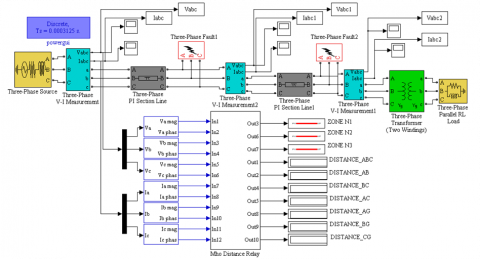Figure 8. 220 kV line simulated on 'SIMULINK MATLAB' with different types of faults with Mho Distance relay

In this part, it seemed necessary to give enough information on the different elements that make up a protection system. These elements are very important, very sensitive and must be well chosen and well-adjusted to ensure effective protection against the different types of anomalies that can occur on the power grid.

Table 2. Power system data and relay setting

 N° Parameters Values 01 Line Longer 100 km 02 Line Voltage 220 KV 03 Frequency 50 Hz 04 Line Resistor $R_{1}$ 0.01165 Ohm/Km 05 Line Resistor $R_{0}$ 0.2676 Ohm/Km 06 Line Reactance $X_{1}$ 0.2725 Ohm/Km 07 Line Reactance $X_{0}$ 0.9495 Ohm/Km Relay Model Parameters N° Zones Parameters Triggered duration (s) 1 First 80 % 0 2 Second 120 % 0.3 3 Third 160 % 1.5

2.6.1 Simulations of short circuit faults in an HTB network with Mho relay protection (220 kV)

Parameter of the line (see appendix)

Direct impedance: ZL=0,011+j0,2752 Ω/km Direct capacity: CL=13,22.10-9 F/km

2.6.2 Simulation N° 1: Remote AG short circuit 70 km with RC-C=10 ohms

In Figure 9, before the short circuit, the voltage Va is equal to the mains voltage. After the appearance of the short circuit (at t=5s), the voltage Va becomes zero. The Iacc short-circuit current is very high (about 10 to 20 rated current). The peak value of the short-circuit current is maximum for the first alternation in the transient regime and then decreases during the following alternations, to stabilize in the permanent short-circuit regime. Figure 10 shows the location of the fault inside the coverage area of the Mho protection relay in Zone 3 at 70 Km.

## 9.1.png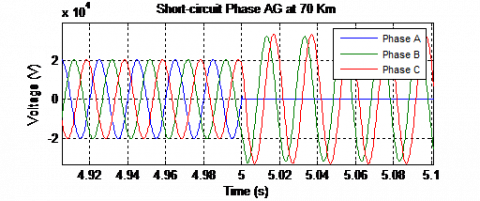## 9.2.png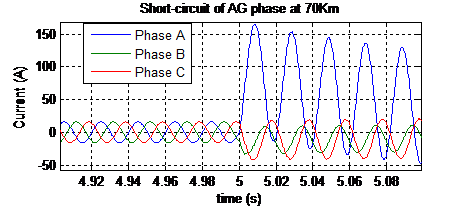Figure 9. Voltage and current signals ABC with fault AG short-circuit at 70Km with Rc-c=10ohms

## 10.pngFigure 10. Location of the AG single-phase fault in the Mho circles: Located at 70 Km inside the coverage area of the Mho protection relay

2.6.3 Simulation N° 2: ABC short-circuit remote 140 km with RC-C=10 ohms

## 11.1.png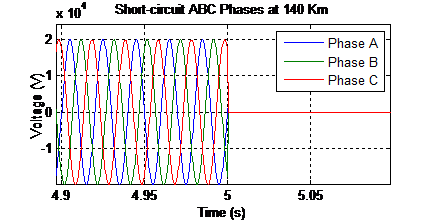## 11.2.png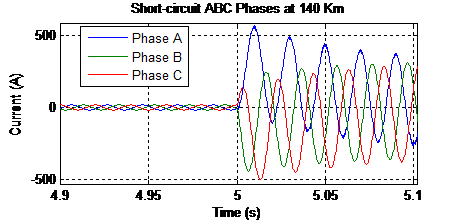Figure 11. Voltage and current signals ABC with AC fault at 140Km with Rc-c=10ohms

## 12.png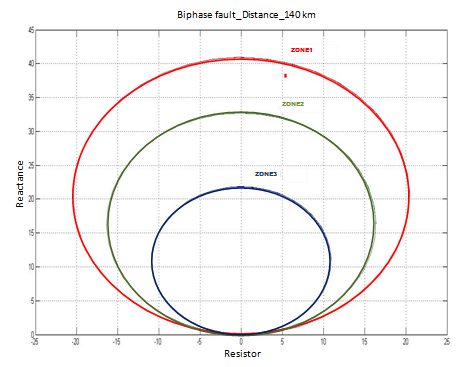Figure 12. Diphase AC fault location in Mho circles: Located at 140 Km inside the Mho protection relay coverage area

In Figure 11, before the short circuit the voltage Va, Vb and Vc is equal to the mains voltage. After the appearance of the short circuit (at t=5s), the voltage Va and Vb becomes zero and the increase of the voltage Vc.

The short-circuit current Iacc and Ibcc is very high (about 10 to 20 the rated current). The peak value of the short-circuit current is maximum for the first alternation in the transient positive value. Figure 12, shows the location of the fault inside the coverage area of the Mho protection relay in Zone 1 at 140 Km.

3. Application of ANN in the Detection of Short-Circuit Currents

Neural networks are mathematical beings, capable of generating behavioral models from input-output data of dynamic systems. Recently, neural networks have been widely used in the modeling, control and monitoring of industrial systems.

In diagnosis, the modeling phase is very important. Indeed, the diagnosis is an aspect that uses the representative model of the process in order to extract the mode of operation in which the process must be located. The use of neural models for measurement, observation and diagnosis puts an end to many of the problems encountered in classical modeling.

3.1 Construction of ANN used and data

The construction of a neuron network is, in a large number of cases, a trial and error process (See Figure 13). To design a high-performance neuron network the parameters relating to both learning (learning rate, algorithm...) and the structure of the neural network must be viewed simultaneously. For learning, there are nuisance parameters such as initial adjustment of synaptic weights, sizes and their ratio for learning and test sets. Good design must be robust to these parameters. In the construction task of the neural network, the adjustable degrees of freedom are:

3.1.1 Type of network

We chose to use the unidirectional layer network and the multilayer feedforward, which is the most widely used network type.

3.1.2 Architecture

A neural network is all the more powerful because it has hidden layers and a high number of nodes in these layers. The sigmoid functions are the most suitable to better approach any nonlinearity.

(1) Number of layers, after several tests, it was retained a 3-layer structure.

(2) Number of neurons per layer a network with several neurons learns better but presents a rather large risk of over learning. The number of neurons in the output layer is fixed by the dimension of the "target" vector which is, in this study, the first hidden layer 55, second hidden layer 7 and the output layer is 7.

(3) Layer activation functions in the hidden layers we used the sigmoid functions and at the output layer a positive unsaturated linear function.

(4) Learning Algorithm: Two gradient retro-gradient algorithms implemented in Matlab's "neural network toolbox" were experimented. It is: the "trainscg" conjugate gradient algorithm: this algorithm is well dedicated to classification problems in the case of large networks and has the advantage of having less memory storage requirement during computation and be fast enough.

The algorithm of the descent of the variable learning rate gradient "traingdx": it converges slowly. Recall that it can sometimes be better to have a slow convergence; this is the case of situations where it is desired to obtain a validation error that is as low as possible.

## 13.pngFigure 13. Installation diagrams of protection with ANN relay

3.2 ANN topology used and data

The topology of the neuronal Mho relay will be represented in Figure 14.

Inputs: The inputs of the network, therefore, are the effective values of voltages and currents and their phase shifts: Va mag, Va phase, Vb mag, Vb phase, Vc mag, Vc phase, Ia mag, Ia phase, Ib mag, Ib phase, and Ic mag, the phase. They are different for each defect.

## 14.png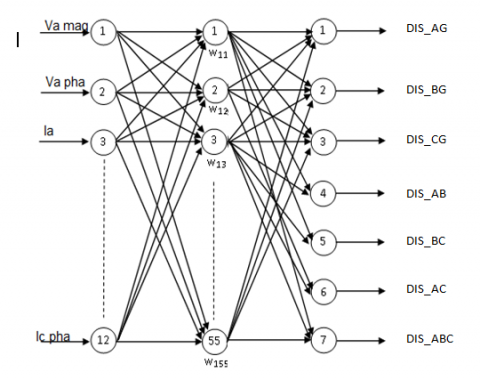Figure 14. Topology of the neuronal Mho relay

Table 3. Learning base including the different short-circuit current faults of the HTB transmission line

 1/ 70 2/ 110 3/ 140 4/ 140 5/ 110 6/ 140 Vs mag 66004 196789 196789 100000 196789 42868.8 Vs phase -10 160 130 -59 60 1.75714 Ia mag 919 128 102 1000 128.096 1120 Ia phase 62.1895 37.1895 -38 -76 76 -88.1895 Vb mag 196789 85994 196789 100000 101606 42868.8 Vb phase 151 -139 -13 -81 -76 -118.243 Ib mag 128 864 892 1000 967 1120 Ib phase -157.81 -220.81 -191.81 119 -98 151.81 Vc mag 196789 196789 94991 197761 101603 42868.8 Vc phase -102 -111 -130 99 -109 121.757 Ic mag 128 128 102 159 967 1120 Ic phase -138 -138 142 100 95 31.8105 Short-circuit faults AG BG CG AB BC ABC

Outputs: The outputs we want to have are the types of defects that occur from the inputs, and that are; the single-phase faults AG, BG and CG, the two-phase faults AB, AC and BC and the three-phase fault ABC.

Hidden layer: The use of a large number of hidden layers is not recommended. The vast majority of classification standards issues use only one or at most two hidden layers. The number of neurons in the hidden layer is usually defined empirically.

Input data: These are the root mean square values of currents and voltages and their phase shifts of the 100 km HTB transmission line. The input data of used in ANN training are organized in Table 3.

3.3 Simulation of detection of short circuit faults by artificial neural networks

This section presents the simulation of Mho's characteristic distance with MATLAB / SIMULINK and the results of the protection tests on the 220kV line and the results of the neuron network fault (short-circuit) simulation and concludes with a comparison between the two methods Classical and ANN) of diagnosis of electrical faults (see Figure 15).

3.4 Simulation results of short-circuit faults on AG and ABC phases

As a result of the defect "a short circuit between phase A & G" which was created voluntarily at time t=5s, it appears that the distance between this last became 71km until the absence of this defect (Figure 16). It is deduced that this defect results between phase A and the earth. It is deduced that this defect is not BG, CG, AB, BC, AC and ABC.

As a result of the defect "of a short circuit between phase A, B & C" which was created voluntarily at time t=5s, it appears that the distance between it has become 140 km until the absence of this said defect (Figure 17). It is deduced that this defect results between the phases A, B and C. It is deduced that this defect is not AG, BG, CG, AB, BC and AC.

## 15.png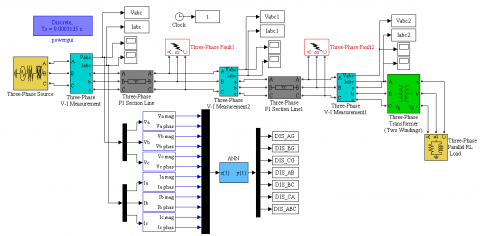Figure 15. Neural digital relay under MATLAB SIMULINK

## 16.png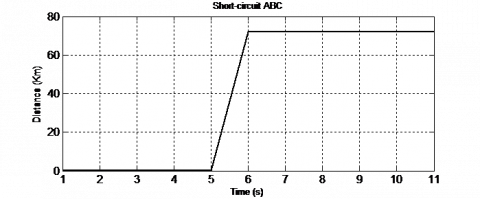Figure 16. Detection of fault case on the line of phase A (distance=70km)

## 17.png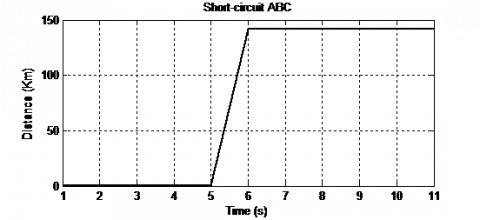Figure 17. Detection of a case of fault on ABC phase lines (distance=140km)

4. Comparison of Results Between the Classical Mho Relay and the Neuronal Mho Relay

After presenting the two types of Mho relays for detection and diagnosis, the application of neural networks in this type of diagnosis, showed their learning ability for the detection of short circuit fault in the transport line of electrical energy, which has provided a better and more accurate detection. In addition, the advantage of artificial neural networks compared to the Mho remote protection relay method, can learn other examples of faults by enriching the initial learning base. In addition, given the generalization capacity of artificial neural networks, they can detect other similar defects in other operating conditions. This method provides speed and reliability in the detection of faults, which consequently gives better maintenance planning, cost minimization to extend the life of electrical network devices.

5. Conclusion

The subject of this work concerns the defects in the electrical transport networks and their location based on measurements of currents and voltages at the source stations. We have shown that the existing fault distance calculation algorithms (Mho relay characteristic and neural network relay). It seemed necessary to give enough information on the different elements that make up a high voltage protection system. These elements are very important, very sensitive and must be well chosen and well-adjusted to ensure effective protection against the different types of anomalies that can occur on the power grid.

Based on distance calculations, we have developed a fault localization algorithm which aims to indicate on a network map the most probable fault location. This system requires knowledge of the topology of the network, and relies on the processing of measurement signals at the source station, which mainly feeds the network. It can be confronted with several difficulties:

• Knowledge of the network topology;

• Exact knowledge of the network parameters;

• The quality of measurements;

•The synchronization of the source post measurements.

With the technological development the old relays (current measuring relays, differential relays, ... etc.) of protection are no longer usable because they have several disadvantages such as lack of precision, delay time in case or break and they only indicate the type of default which is what causes a long duration for the fault location. However, artificial intelligence plays a key role in technological development, and models have been used to simulate a defect using neural networks. The neuron results confirm the simulation results with the Mho features. However, neural networks offer very high benefits, with good accuracy and above all the investment cost is cheaper. The neural relay can be programmed using a PIC (programmable integrated circuit), microcontroller, or a DSP or FPGA that acts as a distance relay.

Finally, the development of Mho distance relays by the neural approach improves the protection of property and localizes defects accurately and better than conventional relays by their response times and their lower costs investment.

Acknowledgment

This work was supported by Electrical Engineering Laboratory (LGE), at the University of Mohamed Boudiaf- M’sila, (Algeria). We like to thank Dr. Djalal Eddine Khodja, and Dr. Salim Chakroune for their helps in the preparing of this paper.

References

 Belwin, J., Edwardk, R. (2018). A review on fault classification methodologies in power transmission systems part-II. Journal of Electrical Systems and Information Technology, 5(1): 48-60. https://doi.org/10.1016/j.jesit.2017.01.004

 Ravikumar, B., Thukaram, D. (2008). Application of support vector machines for fault diagnosis in power transmission system. The Institution of Engineering and Technology, IET Gener. Transm. Distrib, 2(1): 119-130. https://doi.org/10.1049/iet-gtd:20070071

 Soumitri, J., Bhavesh, R., Samantaray, S.R. (2019). A fault zone identification scheme for busbar using correlation coefficients analysis. EEE Power & Energy Society General Meeting (PESGM), Atlanta, GA, USA. https://doi.org/10.1109/PESGM40551.2019.8973764

 Dash, P., Samantaray, R., Ganapati, P. (2007). Fault classification and section identification of an advanced series-compensated transmission line using support vector machine. IEEE Transactions on Power Delivery, 22(1): 67-73. https://doi.org/10.1109/TPWRD.2006.876695

 Patrick, E., Nhlanhla, M., Jan-Harm, C. (2018). Fault detection and location in power transmission line using concurrent neuro fuzzy technique. 18th Annual IEEE Canada Electrical Power and Energy Conference, Toronto, Canada. https://doi.org/10.1109/epec.2018.8598311

 Ripunjoy, P., Rishab, K. (2013). Fault diagnosis of a high voltage transmission line using waveform matching approach. International Journal on Soft Computing (IJSC), 4(4): 33-50. https://doi.org/10.5121/ijsc.2013.4403

 Mehmet, Y., Gokhan, G. (2016). Classification of short-circuit faults in high-voltage energy transmission line using energy of instantaneous active power components-based common vector approach. Turkish Journal of Electrical Engineering & Computer Sciences, 24(3): 1901-1915. https://doi.org/10.3906/elk-1312-131

 Agustín, F., Eduardo, Q. (2014). A modular neural network scheme applied to fault diagnosis in electric power systems. The Scientific World Journal, 176463: 1-13. http://dx.doi.org/10.1155/2014/176463

 Silva, K.M., Souza, B.A. (2006). Fault detection and classification in transmission lines based on wavelet transform and ANN. IEEE Transactions on Power Delivery, 21(4): 2058-2063. https://doi.org/10.1109/TPWRD.2006.876659

 Neeraj, P., Srivastava, R., Vashishtha, K. (2014). Fault detection and isolation (FDI) via neural networks. Journal of Engineering Research and Applications, 4(1): 81-86.

 Xian, T., Zhang, D.P., Wang, Z.H., Liu, X.L., Zhang, H.Y., Xu, D. (2018). Detection of power line insulator defects using aerial images analyzed with convolutional neural networks. IEEE Transactions on Systems, Man, and Cybernetics: Systems, 99(1): 1-13. https://doi.org/10.1109/tsmc.2018.2871750

 Manohar, S., Panigrahi, B.K. (2011). Transmission line fault detection and classification. International Conference on Nagercoil, India. https://doi.org/10.1109/icetect.2011.5760084

 Gowrishankar, M., Nagaveni, P., Balakrishnan, P. (2016). Transmission line fault detection and classification using discrete wavelet transform and artificial neural network. Middle-East Journal of Scientific Research, 24(4): 1112-1121. https://doi.org/10.5829/idosi.mejsr.2016.24.04.23063

 Wei, L., Guo, W. (2010). Waveform matching approach for fault diagnosis of a high-voltage transmission line employing harmony search algorithm. IET Generation, Transmission & Distribution, 4(7): 801-809. https://doi.org/10.1049/iet-gtd.2010.0104

 Peyman, J., Majid, S. (2013). High-frequency transients-based protection of multiterminal transmission lines using the SVM technique. IEEE Transactions on Power Delivery, 28(1): 188-196. https://doi.org/10.1109/TPWRD.2012.2215925

 Bhowmik, P., Purkait, P. (2009). A novel wavelet transforms aided neural network based transmission line fault analysis method. International Journal of Electrical Power & Energy Systems, 31(5): 213-219. https://doi.org/10.1016/j.ijepes.2009.01.005

 Tai, N.L., Chen, J.J. (2008). Wavelet-based approach for high impedance fault detection of high voltage transmission line. European Transactions on Electrical Power, 18(1): 79-92. https://doi.org/10.1002/etep.169

 Yang, C., Yan, H., Neng, T. (2013). A new method of fault phase identification for high voltage transmission lines based on the fault component of phase voltage. Advanced Materials Research, 7325(1): 1056-1064. https://doi.org/10.4028/www.scientific.net/AMR.732-733.1056

 Ahmed, H., Ahmed, Z. (2013). Fault detection and isolation in industrial control valve based on artificial neural networks diagnosis. Control Engineering and Applied Informatics Journal, CEAI, 15(3): 61-69.

 Ren, J., Kezunovic, M. (2012). Teaching protective relaying design and application using new modeling and simulation tools. Journal of Energy and Power Engineering, 6(5): 762-770.

 Yujie, Z., Jimena, L. (2007). Modeling and testing of protection devices for SPS using Matlab/Simulink and VTB, IEEE Electric Ship Technologies Symposium. Arlington, VA, USA, pp. 103-108. https://doi.org/10.1109/Ests.2007.372071

 Soleymani, S., Bastam, M., Mozafari, B. (2013). Fault diagnosis of transmission system based on wavelet transform and neural network. Journal of Intelligent & Fuzzy Systems, 25(2): 271-277. https://doi.org/10.3233/ifs-2012-0634

 Zigler, G. (2008). Numerical distance protection: principles and applications. Third Edition, Publics Corporate Publishing, Berlin - Germany.

 Zellagui, M., Chaghi, A. (2012). Distance protection for electrical transmission line: Equipments, settings zones and tele – protection. Published by LAP Lambert Academic Publishing, ISSN: 978-3-659-15790-5, Saarbrücken - Germany.

 Chuulan, N., Byamvasuren, B. (2007). The fault of electrical transmission lines and function relay protection & automation. International Forum on Strategic Technology, Ulaanbaatar, Mongolia. pp. 221-223. https://doi.org/10.1109/IFOST.2007.4798564

 Ouadi, A., Bentarzi, H. (2016). A new PMU based power swing detector to prevent mal-operation of distance relay. Russian Electrical Engineering, 87(10): 572-578.

 Mini, S., Thomas, A. (2008). Modeling and testing of protection relay IED. International Conference on Power System Technology and IEEE Power India, New Delhi, India, pp. 1-5. https://doi.org/10.1109/ICPST.2008.4745389

 Sidhu, T., Burnworth, J., Darlington, A., Kasztenny, B. (2010). Bibliography of relay literature, 2007 IEEE Committee Report. IEEE Transactions on Power Delivery, 25(1): 88-101. https://doi.org/10.1109/TPWRD.2009.2035388

 Anang, T., Dimas, O. (2015). Optimal coordination of over current relays in radial system with distributed generation using modified firefly algorithm. International Journal on Electrical Engineering and Informatics, 7(4): 691-710. https://doi.org/10.15676/ijeei.2015.7.4.12